Also available in italiano

# The 4-Point backyard diurnal parallax method

Created: 2017-07-27
Author(s):
Eduardo Manuel Alvarez

With this observational, hands-on activity, students can measure the distance of a selected asteroid within an accuracy of 5% in just 2 observational nights, using a telescope, online software and some modest amateur gear. Students will learn that an astronomical observational project requires research, planning and execution and most likely, they will understand that astronomy is one of the few areas where scientific knowledge contribution is still within the reach of serious amateurs.MaterialsGoals
• To demonstrate the Earth’s daily rotation simply
• To learn how to take and process astronomical images
• To learn how to manage observational data
• To determine the distance to an asteroid with acceptable accuracy (about much better than 10%) just by measuring its diurnal parallax displacement as observed from a single location
• To learn how to interpret measurement discrepanciesLearning Objectives
• Explain the effect of diurnal parallax on relative nearby Solar System objects
• Demonstrate the feasibility of measuring accurate astrometric coordinates by means of modest amateur equipment
• Demonstrate that asteroid parallax angles can be measured from just images obtained on two proximate nights – without anything else other than the images!
• Demonstrate valuable scientific data can be obtained that from raw images
• Show that astronomical scientific knowledge is within the reach of serious amateurs, working with modest telescopesBackground

Solar System:
The Solar System consists of the Sun and the objects that orbit the Sun. These include eight planets and various secondary bodies, dwarf planets and small Solar System objects that orbit the Sun directly, as well as satellites (moons) that orbit many planets and smaller objects. The Solar System formed 4.6 billion years ago from the gravitational collapse of a giant molecular cloud. The vast majority of the system's mass is in the Sun, with most of the remaining mass contained in Jupiter. The four smaller inner planets, Mercury, Venus, Earth and Mars, also called the terrestrial planets, are primarily composed of rock and metal. The four outer planets, called the gas giants, are substantially bigger than the terrestrials. The two largest planets, Jupiter and Saturn, are composed mainly of hydrogen and helium. The two outermost planets, Uranus and Neptune, are composed largely of substances with relatively high melting points (compared with hydrogen and helium), called ices, such as water, ammonia and methane, and are often referred to separately as ‘ice giants’. All planets have almost circular orbits that lie within a nearly flat disc called the ecliptic plane.

A solar system refers to a star and all the objects that travel in orbit around it. Our Solar System consists of the Sun - our star - eight planets and their natural satellites (such as our moon); dwarf planets; asteroids and comets. Our Solar System is located in an outward spiral of the Milky Way galaxy.

CCD astro-imaging:
A charge-coupled device (CCD) is an electronic instrument for detecting light. In the case of an astronomical CCD camera, this light is very dim. This has certain implications on how the CCD operates. A CCD uses a thin silicon wafer chip. The chip is divided into thousands or millions of tiny light sensitive squares (or sometimes rectangles) called photosites. Each photosite corresponds to an individual pixel in the final image, and the photosites are often referred to simply as pixels. For clarity in this discussion, ‘photosites’ will refer to the CCD chip and ‘pixels’ will refer to an image. Each photosite is surrounded by a non-conductive boundary, which contains the charge that is collected during an exposure.

Parallax:
Parallax is a method of measuring the distance to an object. Just like how our binocular vision helps us determine distance, the direction to a distant point is slightly different from two separate observation positions. When observing a distant object at exactly the same time from two separated places, if the distance between the observation positions is known, and the angle between them can be measured, with the help of simple geometry, the distance to the object can be calculated. In practice, given that in most astronomical applications, the parallax angle is very small, the only way to take advantage of this technique for distance determination is by means of a very large baseline.

Diurnal Parallax Effect:

Figure 1. Geometry of the diurnal parallax effect. As the Earth rotates, any observer on its surface sees the target’s parallactic angle φ constantly varying, from a maximum value occurring when his Meridian is at right angle to the plane containing the Earth’s rotation axis and the target (some 6 hours prior or after transit time) to a null value whenever the target happens to be placed on his Meridian (target culmination).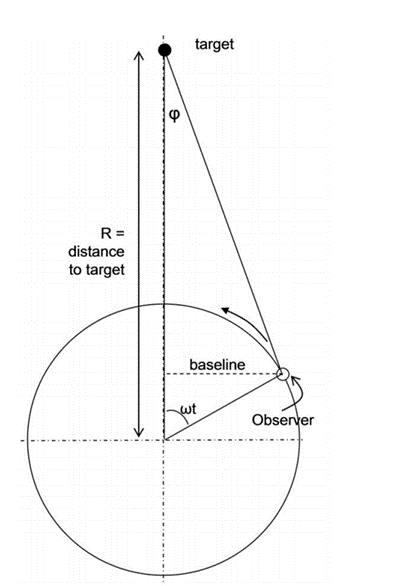Placed on the Earth’s surface, any observer actually moves along a perfectly circular track, several thousand kilometres in radius, making one complete circle each 23.93 hours – the sidereal period. At any given time, the observer is seeing the vault of heaven from a slightly different and continuously changing perspective compared to a fictitious observer placed at rest at the centre of the Earth. The apparent varying displacement of relatively nearby celestial objects with respect to distant ‘fixed’ stars as observed from any ground site, exclusively due to the Earth’s daily rotation, is known as the diurnal parallax effect. This effect materializes as a very subtle angular oscillation, ranging from a few hundreds of arcseconds for sufficiently close near-Earth objects (NEOs) to barely tenths of arcseconds for the furthest observable Solar System objects. The diurnal parallax effect is immeasurably small for celestial bodies beyond the Solar System.

The diurnal parallax effect has nothing to do with the fact that any given Solar System object is moving with respect to the centre of the Earth. Comparatively, the apparent celestial motion of any Solar System body due to its orbital revolution around the Sun becomes orders of magnitude larger than its corresponding diurnal parallax effect. Therefore, the measurement of diurnal parallax must not only deal with minute oscillating displacements with respect to ‘fixed’ stars, but at the same time such tiny effects are always encapsulated within much larger ‘proper motion’ displacements across the sky. Consequently, the first step in the diurnal parallax game requires accurately subtracting the dominant orbital trajectory across the sky from the actually observed apparent celestial motion of the target in question. If carefully and properly done, what remains after this sieving – usually referred to as ‘residuals’ – is only due to the diurnal parallax effect.

Assuming that for a time period as short as a couple of days the distance from the Earth’s centre to the target is basically constant – a generally appropriate assumption except for nearby NEO asteroids and the Moon – the time variability of the parallactic angle can be exclusively attributed to the observer’s changing position relative to a fixed direction in the equatorial plane. In other words, with respect to the plane that contains the axis of rotation of the Earth (i.e. in declination), the parallax angle remains basically invariant. This is why the diurnal parallax is also called the east-west parallax.

The diurnal parallax effect exactly corresponds to the variable parallactic angle – the angle that at any given moment is formed by the target at the vertex, the observer on one side and the centre of the Earth on the other side – projected to the equatorial plane. It’s clear that at the moment of the target’s culmination (when the target happens to be on the observer’s meridian), the variable diurnal parallax angle becomes null, while achieving its maximum theoretical value some 5.98 hours (¼ of sidereal period) either before or after transit. (It would be at exactly ¼ of the sidereal period if the target were not moving with respect to Earth’s centre.)

Therefore, by definition, at any given time t, the variable diurnal parallax angle φ (t) becomes

φ (t) = [RAtopo (t) − RAgeo (t)] cos δ (t) (1)

where RAtopo(t) is the object’s topocentric (measured from the observer location) right ascension at the considered moment, RAgeo(t) is the object’s geocentric (measured from the centre of the Earth) right ascension at the same considered moment and δ(t) is the object’s declination also at the same moment (assumed to be practically the same as measured either from the observer’s place or from the centre of the Earth).

For observations taken before transit, the parallactic projection of the target on the background star field makes it appear to the observer as displaced towards the east with respect to what would be observed at the same time from Earth’s centre – that is, before transit: RAtopo(t) > RAgeo(t). Conversely, the parallactic projection appears displaced towards the west for observations that take place after transit – that is, after transit: RAtopo(t) < RAgeo(t). Therefore, as the Earth rotates, the term RAtopo(t) – RAgeo(t) inside the square brackets continuosly varies as the observer is carried along by the Earth’s rotation, making the variable diurnal parallax angle φ(t) positive for observations taking place before transit, exactly zero at transit, and negative for observations taking place after the transit. Maximum diurnal parallax angles are theoretically reached whenever the observer and the target happen to be placed at right angles with respect to the Earth’s centre.

Meanwhile, taking into account that the target’s declination does vary – gradually – with time but totally independent of the Earth’s rotation, the term cos δ(t) changes only slightly during a short time period of a few days. Therefore, the variable diurnal parallax angle basically varies on a daily basis as a sinusoidal function, which at any time equals RAtopo(t) – RAgeo(t) times the almost constant cosine of the target’s declination, thus becoming:

φ (t) = [RAtopo (t) − RAgeo (t)] cos δ (t) = φmax sin (ω (T0 – t)) (2)

where φmax is the maximum amplitude of the variable diurnal parallax angle for the considered day, ω is the angular sidereal rotation rate of the Earth and T0 is the moment of the target culmination for the considered day. Once φmax is known, the distance D to the target can be readily obtained by making use of the small-angle approximation, thus becoming:

D = RE cos λ / φmax (3)

where RE is the radius of the Earth, and λ is the latitude of the observer (D and RE have to be expressed in the same units, while φmax has to be in radians).

The 4-Point Backyard Diurnal Parallax Method: The 4-Point Backyard Diurnal Parallax Method is a straightforward procedure for determining the key value of φmax from a single station, not requiring any data other than that strictly derived from direct observations. Just by using an amateur-level telescope and CCD on two consecutive nights, imaging continuously for 10 minutes twice each night – at the beginning and at culmination – one can determine φmax accurately, and then use it to compute the distance to the object in question.

The four required data points are the object’s topocentric right ascension measured on two consecutive nights, and corresponding times. On the first night, it should be measured at any time as far as possible from transit (RAtopo (t1)) and at exact transit time (RAtopo (T01)); on the second night, too, it should be measured at any time as far as possible from transit (RAtopo (t2)) and at exact transit time (RAtopo (T02). It doesn’t matter whether the measurements taken in addition to transit each night have the same time difference or not. Therefore, the four required data points actually are four pairs such as: On night #1: (1) RAtopo (t1) and corresponding t1; and (2) RAtopo (T01) and corresponding T01. On night #2: (3) RAtopo (t2) and corresponding t2; and (4) RAtopo (T02) and corresponding T02.

Given that at the moment of transit, the object’s topocentric and geocentric right ascension are exactly the same, a linear approximation for estimating at any other time the otherwise unknown RAgeo (t) can be readily obtained:

(4)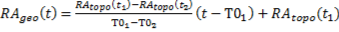This linear approximation for RAgeo (t) helps determine the diurnal parallax angles φ (t1) and φ (t2) measured at times t1 and t2 far apart from transit on each night. On each night, the variation of the diurnal parallax angle can be represented by a sinusoidal curve that, besides becoming null at the transit time, happens to pass through the measured φ (t) point. Such sinusoidal curves are unique; therefore once such curves are found, the maximum amplitude of the variable diurnal parallax angle for that day (respectively, φmax1 and φmax2) is known. Considering that both φmax1 and φmax2 are derived from a linear approximation that necessarily makes one of them underestimated and the other overestimated (a happy circumstance duly explained in correspondent published papers), an accurate final φmax value results from averaging them:

φmax (φmax1 + φmax2) (5)

Figure 2: Opposition - The apparent motion in right ascension of a minor planet. Most of the time it displaces towards higher RA values (direct motion) but once a year, as it approaches the closest distance to Earth, it appears moving backwards to lower RA values for a while (retrograde motion).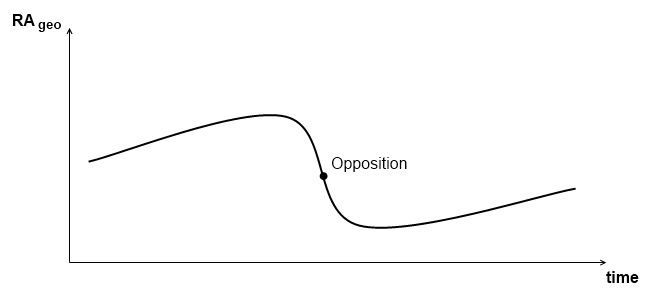Figure 3: Before Opposition - The real apparent retrograde motion in RA of a minor planet before opposition. The instant speed continues to increase until opposition is reached.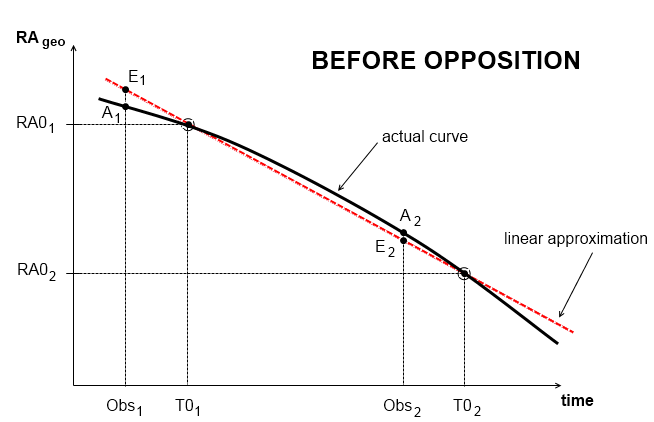Figure 4: After Opposition - The real apparent retrograde motion in RA of a minor planet after opposition. The instant speed continues to decrease until the loop is ended and direct motion reassumed.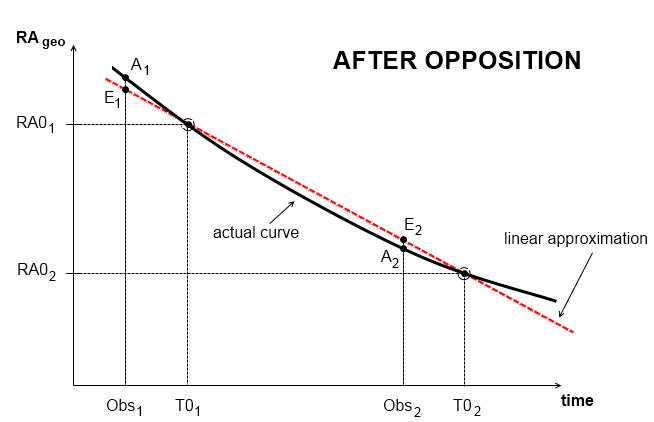Full Description

### Step 1: Selecting an appropriate target

Students have to research asteroids appropriate for this project. Potential targets for this project are the main-belt asteroids that at the time of observations will be relatively close to opposition, appearing relatively high on the local eastern skies at dusk. Of those appropriate candidates, the brighter the better.

### Step 2: Taking the required images on night 1

In order to improve accuracy, in practice the required 4 data points will be obtained not from just 4 single isolated observations but instead from 4 sets of images – 2 sets on the first night, and another 2 sets on the following night. On each night, the first set will be obtained soon after dusk sky turns dark enough, and the second set around the target’s transit time.

Students should have the telescope and the imaging system duly prepared at least 15-minute before dusk. That is, for the telescope, the students should ensure that it is properly polar aligned, its pointing position is accurately known and that the tracking system operates normally. With the imaging system, the students should check that the CCD camera is correctly connected to its hardware control, it has achieved and stabilized at the operating temperature, the imaging software is running normally, the carpet where the images are to be placed has been defined, and the proper name/number designation of each image to be captured has been set. For asteroid targets brighter than 14 magnitude, to be imaged by means of a 20-cm telescope using a decent tracking system, exposure times should not be longer than 30 sec, so that no autoguiding is actually required.

The next step is to get the accurate time. The easiest way to do it is by synchronizing the PC running internal-time to an Internet atomic-time provider.

The first task to do after the first bright stars appear on the sky is to focus the camera. A Bahtinov mask helps to do so in a simple and straightforward way.

After having successfully focused the camera, the next step is to point the telescope to the target and place it around centre of the CCD frame. The easiest way to do it is by means of an accurate pointing system, just directing the telescope to slew to the target’s equatorial coordinates.

The asteroid in question must be recognized within the CCD frame. The easiest way to do it is by taking an image and immediately running it on a software tool that automatically detects its astrometric position and photometric value. The first one will help ensure that the images have captured the target; the second one will ensure that the selected exposure time helps achieve a proper signal/noise ratio (for the required astrometric goal, a SNR as low as 20 will be enough).

After verified the imaging requirements, now it’s time to continuously image the asteroid target for about 10-12 minutes. There is no constraint on the exact time this set of images should be actually taken – although preferably it should be as far apart from the transit time as possible. This is the set of images from where the first data point of the required ‘4-Point’ will be obtained after processing. After this set of images is taken, there is nothing more to do until about 30 minutes before the transit time.

At about 30 minutes before the target’s transit time (i.e. when its right ascension exactly matches local sidereal time), it’s time to once again prepare for the second set of images. The stepwise list is exactly the same as before.

After verifying all the imaging requirements, it’s time to continuously image the asteroid target for about 10-12 minutes around the transit time. In contrast to the first set, the imaging interval of this set has to inevitably match the transit time – that is, it is imperative that some images are taken prior to the transit time, and other images are taken after the transit time. This is the set of images from where the second data point of the required ‘4-Point’ will be obtained after processing. After this set of images is taken, there is nothing more to do.

### Step 3: Taking the required images on night 2

On the following night (‘Night 2’), the activities to be performed are exactly the same as on ‘Night 1’. The asteroid target has to be continuously imaged for about 10-12 minutes as far apart from the transit time as possible, and for about another 10-12 minutes around the transit time. These sets of images will be the sources for the third and fourth data points of the required ‘4-Point’.

### Step 4: Processing images and acquiring the required data

Students have to process the captured images by performing due reduction and astrometry. By applying a proper astrometric software tool to each taken image, the corresponding target’s topocentric right ascension can be obtained (one value per image). After discarding any suspiciously wrong value, the four topocentric right ascension data points can be determined by averaging the obtained topocentric right ascension values corresponding to each set of images.

Once the required four topocentric right ascension values are obtained, all that remains is determining the right time corresponding to each obtained value.

The right times (t1 and t2) associated with the topocentric right ascension values derived from the two sets far from transit are non-critical; thus, they can be obtained just from averaging the times of the corresponding images. However, the two transit times (T01 and T02) are critical – even a difference of a few seconds is important – so that they must be determined precisely by making careful use of the data around the transit on each night.

The easiest way to find the precise transit time for each night is by using a spreadsheet. The rows contain data corresponding to each image around transit, while the column headings consist of (1) the obtained target’s topocentric right ascension value, (2) the image’s time, and (3) the corresponding local sidereal time (expressed in the same units as the topocentric right ascension values). Then, in a fourth column, the local sidereal time will be subtracted from the target’s topocentric right ascension value. Finally, the exact transit time will be calculated by making use of the intercept function applied to data in columns (2) and (4).

### Step 5: Computing data

Once the four data points [RAtopo(t1), t1], [RAtopo(T01), T01], [RAtopo(t2), t2], [RAtopo(T02), T02] are known, proper manipulation according to the equations of the 4-Point Backyard Diurnal Parallax Method is all that is required.

From equation (4), the linear approximation for finding out RAgeo at any time becomes known, so that from equation (1), the diurnal parallax angle φ at times t1 and t2 becomes known. Once φ(t1) is known, from equation (2), the maximum amplitude of the variable diurnal parallax angle for the first night (φmax1) can be readily obtained, with the help of

φ (t1) = φmax1 sin (ω (T01 – t1))

Similarly, the maximum amplitude of the variable diurnal parallax angle for the second night (φmax2) can be computed from

φ (t2) = φmax2 sin (ω (T02 – t2))

Finally, from equation (5), the key value φmax becomes known, so that from equation (3), the distance to the asteroid is determined.

Step 6: Assessing the quality of the found distance Students have to compare their outcome with the published values. Analysis of possible error sources and their relative importance should be critically discussed.

Step 7: Writing a formal report The activity should conclude with the writing of a succinct scientific report on the determined asteroid distance and its comparison with actual distance, along with a critical discussion about the potential sources (and their corresponding relative weights) of found discrepancy and ways to minimize them in further attempts.Evaluation
• Students should make a plan the due observations at field. Ask for a brief explanation for the selected asteroid target they are about to work with. Ask how they are going to precisely obtain the time of each one of the images.
• Students should obtain astrometric coordinates from their images. Ask how they are going to accomplish this. Ask them about the expected accuracy.
• Students should design and use a proper spreadsheet to manage their obtained astrometric data. They should perform the numerous unit conversions carefully.
• Students should conclude with a report of the found asteroid distance and its comparison with the actual distance, along with a critical discussion about the potential sources of found discrepancy. Ask about the corresponding relative weights of each source of error, and how to possibly minimize their effect on further attempts.
• Students should be able to discuss and provide a brief explanation for the following questions: o Why the year-long apparent displacement of asteroids in the sky is as it is? o Why is it convenient to observe asteroids near their opposition? o Why the observer’s latitude becomes important to the diurnal parallax effect? o What is the difference between topographic and geocentric coordinates? o Why is the 4-Point simplification for determining the asteroid’s parallax effective? o How can astrometry data be properly obtained from an astronomical image?Additional Information

Two related published papers:

Diurnal Parallax Determinations of Asteroid Distances Using Only Backyard Observations from a Single Station http://adsabs.harvard.edu/abs/2012SASS...31...45A

The 4-Point Backyard Diurnal Parallax Method Tested on a Challenging Far Away Target: Dwarf Planet 134340 Pluto http://adsabs.harvard.edu/abs/2013SASS...32...25A

Two related PPTs: (in English): Diurnal Parallax Determinations of Asteroid Distances Using Only Backyard Observations from a Single Station http://www.olasu.com.uy/contenidos.php?action=detalle&idmenu=12&idcontenido=117

(in Spanish): Nuevo y Simple Método para la Medición del Paralaje Diario de Cuerpos Menores del Sistema Solar, requiriendo solo cuatro datos a partir de imágenes obtenidas desde un único lugar http://www.olasu.com.uy/contenidos.php?action=detalle&idmenu=12&idcontenido=116

Author’s website: http://www.olasu.com.uy